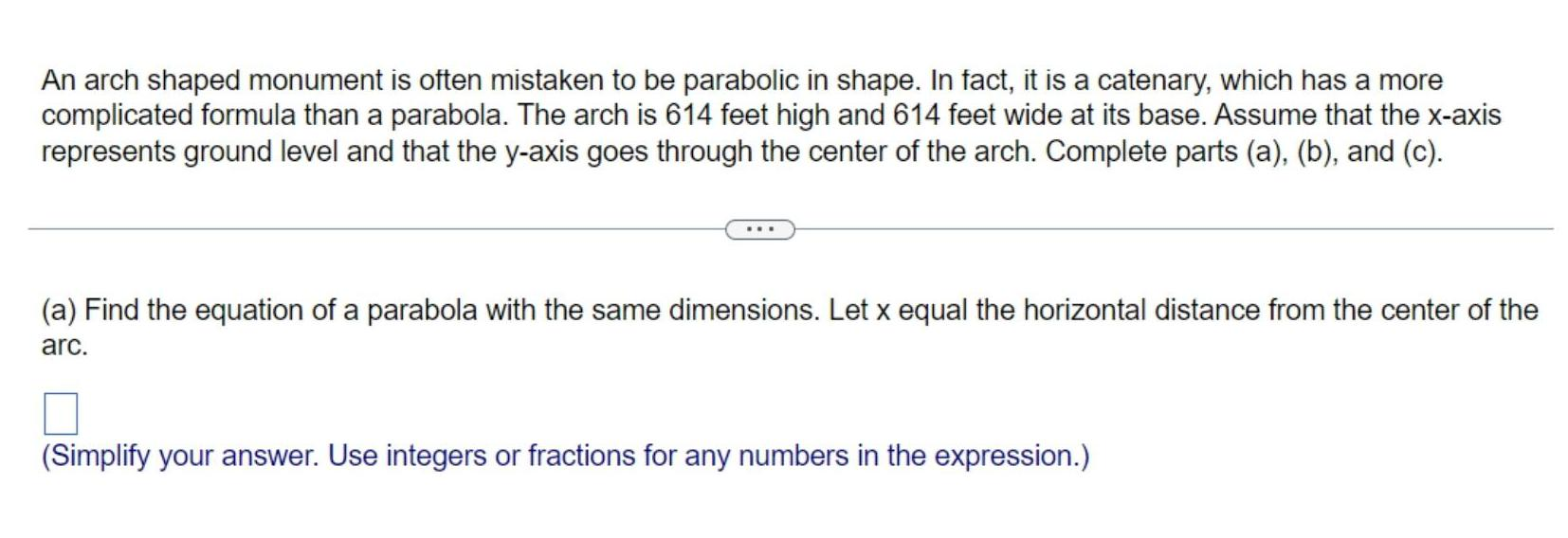Question:

# An arch shaped monument is often mistaken to be parabolic in

Last updated: 11/18/2023An arch shaped monument is often mistaken to be parabolic in shape In fact it is a catenary which has a more complicated formula than a parabola The arch is 614 feet high and 614 feet wide at its base Assume that the x axis represents ground level and that the y axis goes through the center of the arch Complete parts a b and c a Find the equation of a parabola with the same dimensions Let x equal the horizontal distance from the center of the arc Simplify your answer Use integers or fractions for any numbers in the expression